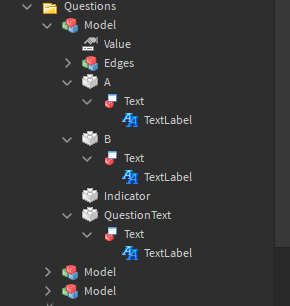# Issue with for loop

Hello, I’m trying to make a script that randomizes the questions and answers in models. Having some issues here. It instead renames one of them 3 times and then moves to the next one.
https://gyazo.com/ced66417b709c4482ec181da6313ff1f
The correct way would be, that it would change the text on each one with different text.

Snippet of the script:

``````local Folder = game.Workspace.Questions

local questions = {"Test 1 question", "Test 2 question", "Test 3 question"}
local A_Claims = {"Test A1 claim", "Test A2 claim", "Test A3 claim"}
local B_Claims = {"Test B1 claim", "Test B2 claim", "Test B3 claim"}
local CorrectAnswers = {"A", "B", "B"}
wait(5)
for x, Model in pairs(Folder:GetChildren()) do
local Question = Model.QuestionText.Text.TextLabel
local A_Claim = Model.A.Text.TextLabel
local B_Claim = Model.A.Text.TextLabel
for index in ipairs(questions) do
Question.Text = questions[index]
wait(1)
end
end
``````

Configuration:Any help is appreciated, thanks!

You’re basically getting all the index in the questions and renaming it with all the index in the table each second, right now i have to go but you can use math.random() to find a random one in the table but since i don’t know how to do it you must figure it out.

Since i have more time, you can check if the script got the third question then getting the 3rd index in the tables in order so it won’t bug out.

1 Like

I have solved the issue by using math.random() and table.remove().
For those that may stumble on this someday:

``````local Folder = game.Workspace.Questions

local questions = {"Test 1 question", "Test 2 question", "Test 3 question"} -- table
wait(5)
for x, Model in pairs(Folder:GetChildren()) do
local Question = Model.QuestionText.Text.TextLabel -- Textlabel to edit

local random = math.random(#questions) -- Get random number from 1 to amount of options in table
Question.Text = questions[random] -- Change the text in random string.
table.remove(questions, random) -- Remove random from table to prevent duplicates
end
``````